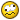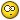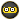# Mechanics A2 help needed

#### xcalibur

##### Gbatemp's Chocolate Bear
OP
Member
I did a Mechanics A2 maths exam and I think I did fairly well on it.
After the exam all of my friends got together and we kind of discussed it. One particular question which they all didn't get but I did is bugging me now. They said that I did it wrong but any way I look at it, I'm right.
Thats why I want to give you the question and method of answering so you can decide wether I'm right or wrong...

First some values;

mass of P = 0.5 kg
Modulus of Elasticity = 20 N
Natural Length = 1.25 m

A light elastic string is connected to point O at one end and partice P to another.
When velocity of the particle = V;

i) Show that V^2= -32X^2+20X+25
ii) Find the maximum velocity
iii) Find the acceleration when the particle is at the lowest point.

i) For this question I just equate the energy at O right before the particle is dropped. This is only Potential energy because there isn't any extension or velocity when P is at O. This means mgh = (0.5)(10)(1.25+x).
Then I equate the energy at any point during the drop. Because this new position is taken as the lowest level, there is no Potential energy. Only Kinetic and Elastic Potential energy(if the particle dropped lower than 1.25 m).
This means that KE+EPE= 0.25V^2 + 8X^2. Initial energy = Final energy -> and the rest is all algebra.

ii) I assumed that the maximum velocity is at the equilibrium position.
I assume this because, when velocity is maximum, acceleration is zero.
And according to Newtons law; Forces with the motion - forces against the motion = mass x acceleration.
So this means that T=MG since when its moving, the resisting force is the tension and the driving force is the weight.
I just worked out the extension at the equilibrium position by substituting T = (Modulus of Elasticity x Extension)/Natural Length
That way I can find the extension at this point and just substitute it into the equation found earlier.

iii) This was done by equating energies like described before but now there isn't any kinetic energy since the particle is at the lowest point and therefore has stopped moving. I find the extension at this point by just equating energies again.
Then I use Newtons law, which in this case looks like this; T - MG = MA. Then I just find the accelleration.

This one question bothered me really badly so please tell me I did this right...
My friend said that the maximum speed isn't at the equilibrium position so if he's right, this messed up most of the question since part ii had the most marks.
If I got this right, I'm pretty sure that I've aced it.
Btw, this isn't the final question. The final question was a simple question to do with motion under variable forces. Lots of integration/differentiation (though not a lot when compared to the pure) and you get a shit load of marks for only about 5 lines of work.

#### xcalibur

##### Gbatemp's Chocolate Bear
OP
Member
No one?
I didn't get uch sleep last night because of this and I know its gonna keep nagging at me.
NO one here has taken A2 mechanics?

I wanted to ask my teacher but he refused and said he wanted to focus on the pure maths exam coming up in 2 days...

#### Lee79

MemberHope you pass though good luck.

#### moozxy

##### hamboning
Member
I'm doing A2 but instead of doing M1 and M2 or S1 and S2, I did M1 and S1, so can't help you lol.

C2 on Monday? I'm resitting C2 and C3, then we got C4 on next next thursday -.-
What board are you sitting?

#### xcalibur

##### Gbatemp's Chocolate Bear
OP
Member
moozxy said:
I'm doing A2 but instead of doing M1 and M2 or S1 and S2, I did M1 and S1, so can't help you lol.

C2 on Monday? I'm resitting C2 and C3, then we got C4 on next next thursday -.-
What board are you sitting?

Cambridge.
I got paper 3 (pure A2) next week and I'm nervous as hell.
Although I should've taken M1 and S1, my physics teacher told me that it'd be better if I took M2 seeing as I'm doing physics A2.

#### Veho

##### The man who cried "Ni".
Former Staff
Could you draw the setup, please? Is point 0 the same as the equilibrium point? Does the first question require a general equation or is there a set of initial conditions?

(And can we use integrals? I like integrals...)

EDIT: Is point 0 the natural length of the elastic string?

#### xcalibur

##### Gbatemp's Chocolate Bear
OP
Member
Veho said:
Could you draw the setup, please? Is point 0 the same as the equilibrium point? Does the first question require a general equation or is there a set of initial conditions?

(And can we use integrals? I like integrals...)

EDIT: Is point 0 the natural length of the elastic string?

I made a crappy drawing of the setup so I hope you get it.

[The first one is where the particle (P) is at O. O is connected to the ceiling btw, I'm sorry if that wasn't clear.
The natural length is 1.25 m.
The second part is when its falling and the equation described in part i can be found by taking the first part as the initial position and the second part as the final position, where the extension of the wire and the velocity at this point are unknown.
The last part is at the lowest point where only the extension is unknown so I can find it by taking the first part as the initial position and the lowest position as the final.

Sadly this question doesn't have any integrals. The final question was nice though.

#### Veho

##### The man who cried "Ni".
Former Staff
Are we to assume that until the particle reaches the natural length of the string, the string does not affect the particle (i.e. the particle is in free fall?)

i) I think this one is okay.

ii) The maximum velocity is when the total acceleration equals zero, a1 = a2, meaning 10 = (x - 1.25) * 40. After that point, the elastic force exceeds the gravitational pull and the particle starts slowing down.

x = 1.25 + 10/40 = 1.25 + 0.25 = 1.5 m.

You can get the Vmax from the equation you had to prove in i) and the x you get here.

iii) Yes, you equate energies, the elastic potential energy at the lowest point is equal to the maximum kinetic energy, at maximum V. You have the Vmaxfrom ii). You end up with Vmax^2, and Ekinetic max = Vmax^2*m / 2
Ekinetic = V^2*m / 2

The elastic potential energy at the lowest point is defined as xlowest^2 * Me / 2.

Me = modulus of elasticity.

Now equate the two and find X. Then, a = F / m, and F = Me * {elongation from natural length}, in this case (x - 1.25).

I this is a correct procedure. Do the math, see what this gets you, and compare the results to the ones you got.

Of course, I might be wrong.

#### xcalibur

##### Gbatemp's Chocolate Bear
OP
Member
Veho said:
Are we to assume that until the particle reaches the natural length of the string, the string does not affect the particle (i.e. the particle is in free fall?)

i) I think this one is okay.

ii) The maximum velocity is when the total acceleration equals zero, a1 = a2, meaning 10 = (x - 1.25) * 40. After that point, the elastic force exceeds the gravitational pull and the particle starts slowing down.

x = 1.25 + 10/40 = 1.25 + 0.25 = 1.5 m.

You can get the Vmax from the equation you had to prove in i) and the x you get here.

iii) Yes, you equate energies, the elastic potential energy at the lowest point is equal to the maximum kinetic energy, at maximum V. You have the Vmaxfrom ii). You end up with Vmax^2, and Ekinetic max = Vmax^2*m / 2
Ekinetic = V^2*m / 2

The elastic potential energy at the lowest point is defined as xlowest^2 * Me / 2.

Me = modulus of elasticity.

Now equate the two and find X. Then, a = F / m, and F = Me * {elongation from natural length}, in this case (x - 1.25).

I this is a correct procedure. Do the math, see what this gets you, and compare the results to the ones you got.

Of course, I might be wrong.

Sigh... That put me to rest.
We couldn't BOTH do it wrong right?

#### Extreme Coder

##### Well-Known Member
Member
Sorry, can't help you with that at all, I will be taking A2 next year :/

D
•Replies
0
Views
1K
D
D
•Replies
1
Views
1K
•Replies
21
Views
5K
D
Replies
7
Views
1K
D
•Replies
6
Views
2K

### Recent Content

12. #### Criminal Girls 2 JP With DLC

Help Users
• No one is chatting at the moment.